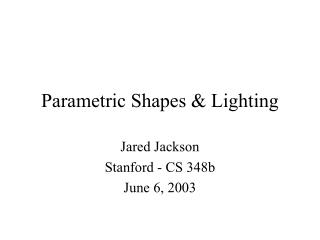DownloadDownload PresentationParametric Shapes & Lighting

# Parametric Shapes & Lighting

Télécharger la présentation## Parametric Shapes & Lighting

- - - - - - - - - - - - - - - - - - - - - - - - - - - E N D - - - - - - - - - - - - - - - - - - - - - - - - - - -
##### Presentation Transcript

1. Parametric Shapes & Lighting Jared Jackson Stanford - CS 348b June 6, 2003

2. Shapes from Parametric Paths • A parametric path in multiple dimensions requires only one variable • Circle: u -> 0 to 1x(u) = sin(2 pi u), y(u) = cos(2 pi u), z(u) = 0

3. Shapes from Parametric Paths • Mapping a 2D path along the 3D path gives a 3D parametric shape • For a torus, trace a circle along a parametric path • This requires that we know the normal to the path

4. shapes/parametric.cc • Create a shape using S-Expressions for • x, y, z • dx, dy, dz • Ex: sin (2 pi u) -> (sin (mult 2 (mult pi x))) • Other parameters include: • Radius of the 2D shape • Twist angle of the 2D shape • Min and max of u • Number of samples to take along u

5. Parametric Torus Surface “parametric” “x” “mult 2 (cos (mult 2 (mult x pi)))” “y” “mult 2 (sin (mult 2 (mult x pi)))” “z” “0” “dx” “mult -1 (sin (mult 2 (mult x pi)))” “dy” “cos (mult 2 (mult x pi))” “dz” “0” “radius” “0.3” “samples” 20 “min” 0 “max” 1

6. Other Parameters: Shapes • There are several built-in 2D shapes: • Circle (tube) • Square (box, disc) • Star • And more

7. Other Parameters: Complex Shapes • Shapes can also be described as a 2D parametric path using S-Expressions “shape” “complex” “cx” “sub 1 (pow x 3)” “cy” “x” “csamples” 20

8. Other Parameters: Radius • The radius is a scaling factor on the 2D shape that can also be specified as an S-Expression “radius” “0.2” “radius” “add 0.3 (mult 0.1 (cos (mult 2 (mult x pi))))”

9. Other Parameters: Twist • The twist parameter rotates the 2D shape within its plane before mapping it along the path “twist” “cos (mult 2 (mult x pi))”

10. Basket Weaving • x(u) = (r1) * cos(2 pi u) • y(u) = 0.75 * u • z(u) = (r1) * sin(2 pi u) • radius(u) = 0.35

11. Parametric Lights • Lights can also follow a 3D parametric path • The sample points then act as point light sources • Light intensity is divided across the number of sample points

12. Parametric Lights

13. A Final Image